Six-sided polygon

In a six-sided polygon. The first two angles are equal, the third angle is twice (the equal angles), two other angles are trice the equal angle, while the last angle is a right angle. Find the value of each angle.

Leave us a comment of example and its solution (i.e. if it is still somewhat unclear...):Be the first to comment!To solve this verbal math problem are needed these knowledge from mathematics:

Do you have a system of equations and looking for calculator system of linear equations? See also our trigonometric triangle calculator.

Next similar examples:

1. In a 2In a thirteen sided polygon, the sum of five angles is 1274°, four of the eight angles remaining are equal and the other four are 18° less than each of the equal angles. Find the angles. .
2. Rotatable tower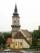Rotatable tower situated in the city center has ground shape of a regular polygon. If the tower is rotated by 14.4° around its centerpiece it looks from the side same. Your task is to calculate at least how many vertices can have a ground plan view of the.
3. Largest angle of the triangleWhat is the largest angle of the triangle if the second angle is 10° greater than twice the first and the third is 30° smaller than the second?
4. Triangle anglesIn a triangle ABC the interior angle at the vertex C is twice as the internal angle at the point A. Outer angle at the point B measured 117 degrees. How big is the outer angle at the vertex A?
5. AnglesThe triangle is one outer angle 158°54' and one internal angle 148°. Calculate the other internal angles of a triangle.
6. AnglesIn the triangle ABC, the ratio of angles is: a:b = 4: 5. The angle c is 36°. How big are the angles a, b?
7. Internal angles IST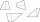Determine internal angles of isosceles trapezium ABCD /a, c are the bases/ and if: alpha:gamma = 1:3
8. Trapezium internal anglesA trapezium where AB is parallel to CD, has angle A : angle D = 4 :5, angle B = 3x-15 and angle C = 4x+20. Find angle A, B, C and D.
9. 9-gon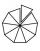Sum of interiol angles of 9-gon is:
10. Angles 1It is true neighboring angles have not common arm?
11. Hexagon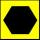Draw a regular hexagon inscribed in a circle with radius r=8 cm. What is its perimeter?
12. DiagonalCan a rhombus have the same length diagonal and side?
13. Angle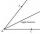Draw angle |∠ ABC| = 130° and built its axis. What angle is between axis angle and arm of angle?
14. Center traverseIt is true that the middle traverse bisects the triangle?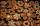John decorated 29 gingerbread and Annie 8 more. How many gingerbread decorated Annie?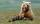Winnie Hugo had the right paw 10 punches and on 15 left more. How many punches has on left paw?Solve two equations with two unknowns: 400x+120y=147.2 350x+200y=144